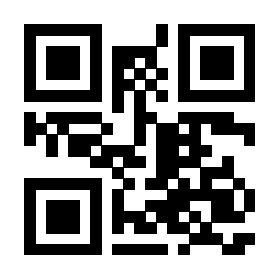# 如何在目录中插入文字啊？？

\documentclass{ctexbook}
\usepackage[toc]{multitoc}
\setlength\columnsep{3em}
\begin{document}
\tableofcontents
\chapter{标题}
\chapter{摘要}
\part{第1部分}
\section{第1节}
\section{第1小节}
\section{第2小节}
\part{第2部分}
\section{第1节}
\end{document}0

1.3k
3 个回答

\addtocontents{toc}{\protect\begin{multicols}{2}}
\part{第一部分}

\AtEndDocument{\addtocontents{toc}{\protect\end{multicols}}}\documentclass{ctexbook}
\usepackage{multicol}
\setlength\columnsep{3em}
\begin{document}
\tableofcontents
\chapter{标题}
\chapter{摘要}
\part{第1部分}
\section{第1节}
\section{第1小节}
\section{第2小节}
\part{第2部分}
\section{第1节}
\end{document}

### 撰写答案